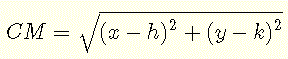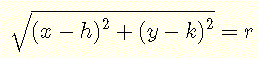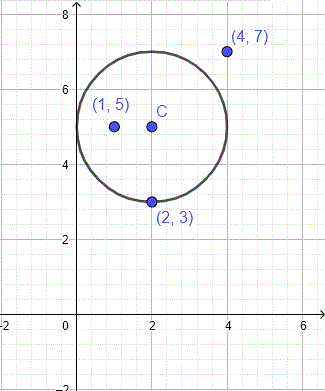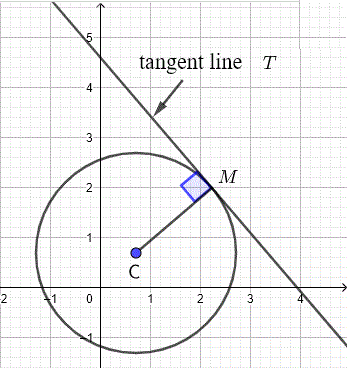# Equation of a Circle

Page Content

## Equation of a Circle in Standard Form

A circle with center C given by its coordintaes C(h,k) is shown below. By definition, all points M(x,y) on the circle are at equal distance from the center. In other words, a circle of center C is the set of all points that are at equal distance from point C . This distance between C and any point on the circle is called the radius and has length r in the graph below.The distance from the center C(h,k) to a point M(x,y) on the circle is given byTo find the equation, we use the definition to write that distance CM is equal to the radius rWorking with square root add extra difficulties that can be avoided. The square root in the above equation may be eliminated by squaring both sides of the equation to obtainSimplify to obtain the standard equation of a circle with center C(h,k) and radius r
    $(x - h)^2 + (y - k)^2 = r^2$

## Examples

Example 1
Find the standard equation of the circle with center C(2,5) and radius r = 2

Solution to Example 1
Given the center and the radius, the standard equation of the circle is given by:
$$(x - 2)^2 + (y - 5)^2 = 2^2$$

## Points on, Inside or Outside a Circle

To find out if a given point is on a circle, inside a circle or outside a circle, we compare the square of the distance from the center of the circle to the given point to the square of the radius. We use the square of the distance instead of the distance to avoid using the square root.

For a circle of center $$C(h,k)$$ and radius $$r$$, point $$P$$ with coordinates $$(x_0 , y_0)$$
1) is on the circle, if the following equality is satisfied:
$(x_0 - h)^2 + (y_0 - k)^2 = r^2$
2) is inside the circle, if the following inequality is satisfied:
$(x_0 - h)^2 + (y_0 - k)^2 \lt r^2$
3) is outside the circle, if the following inequality is satisfied:
$(x_0 - h)^2 + (y_0 - k)^2 \gt r^2$

Example 2
Equation of a circle and points inside, outside or on the circle
Which of the following points $$P_1(1,5)$$ , $$P_2(2,3)$$ and $$P_3(4,7)$$ is inside, outside or on the circle given in example 1?

Solution to Example 2
The center of the circle in example 1 is at $$C(2,5)$$ and has radius $$r = 2$$
Find the square of the distance from the center of the circle to each of the given point and compare it to the square of the radius
square of distance from $$C$$ to $$P_1$$ is given by: $$(2-1)^2 + (5-5)^2 = 1$$ which is smaller than $$r^2 = 4$$. Hence point $$P_1$$ is inside the circle.
square of distance from $$C$$ to $$P_2$$ is given by: $$(2-2)^2 + (5-3)^2 = 4$$ which is equal to $$r^2 = 4$$. Hence point $$P_1$$ is on the circle.
square of distance from $$C$$ to $$P_3$$ is given by: $$(2-4)^2 + (5-7)^2 = 8$$ which is larger than $$r^2 = 4$$. Hence point $$P_3$$ is outside the circle.
The circle and the three points are shown below and we can easily check the answer found above.## Tangent line to a circle

One of the important properties of a tangent line to a circle is that it is perependicular to the line through the center $$C$$ and the point of tangency $$M$$ as shown below.Example 3 Equation of a circle and points inside, outside or on the circle
Find the equation of the tangent line to the circle with equation $$(x + 2)^2 + (y - 2)^2 = 5$$ at the point $$M(-4 , 3)$$.
Solution to Example 3
Comparing the given equation to the general standard equation given above, we deduce that the center is at $$C(-2,2)$$.
The slope $$m_1$$ of the line through $$C M$$ is given by
$$m_1 = \dfrac{3-2}{-4-(-2)} = -\dfrac{1}{2}$$
Let $$m_2$$ be the slope of the tangent line. Since the tangent line and $$C M$$ are perpendicular, the slopes $$m_1$$ and $$m_2$$ are related by
$$m_1 \times m_2 = -1$$
which gives
$$(-\dfrac{1}{2}) \times m_2 = -1$$
solve for $$m_2$$ to obtain
$$m_2 = 2$$
we now know the slope $$m_2$$ and $$M(-4,3)$$ the point of tangency through which the tangent line passes, we use the point slope formula to find the equation of the line tangent to the given circle
$$y - 3 = 2 (x - (-4))$$
$$y = 2 x + 11$$
As an exercise, plot the given circle and the equation of the tangent line found above and check graphically that they are tangent at the point (-4,3).

## General Form of the Equation of a circle

$$(x - h)^2 + (y - k)^2 = r^2$$
Expand
$$x^2 - 2 h x + h^2 + y^2 - 2 k y + k^2 = r^2$$
Let
$$A = - 2 h$$, $$B = - 2 k$$ and $$C = h^2 + k^2 - r^2$$
Substitute in the expanded equation to obtain the general form of the equatin of a circle.
$$x^2 + y^2 + A x + B y + C = 0$$

Example 4 Find the equation of a circle given three points
Find the equation of the circle through the points $$P_1(6,4)$$, $$P_2(-1,5)$$ and $$P_3(2,-4)$$.
Solution to Example 4
The coordinates of a point on a circle must satisfy the equation of the circle. We write that the coordinates of each of the given points satisfy the equation of the circle in the general form: $$x^2 + y^2 + A x + B y + C = 0$$.
Point $$P_1(6,4)$$ is on the circle; substitute $$x$$ by $$6$$ and $$y$$ by $$4$$ in the equation, hence: $$6^2 + 4^2 + 6 A + 4 B + C = 0$$
Point $$P_2(-1,5)$$ is on the circle; substitute $$x$$ by $$-1$$ and $$y$$ by $$5$$ in the equation, hence: $$(-1)^2 + 5^2 - A + 5 B + C = 0$$
Point $$P_3(2,-4)$$ is on the circle; substitute $$x$$ by $$2$$ and $$y$$ by $$-4$$ in the equation, hence: $$2^2 + (-4)^2 + 2 A - 4 B + C = 0$$

Solve the for (A,B,C) the system of equations obtained above and shown below in standard form
$$\begin{cases} 6 A + 4 B + C = -52\\ - A + 5 B + C = - 26 \\ 2 A - 4 B + C = -20 \end{cases}$$

Use any method to obtain the solution
$$A = -4$$ , $$B = -2$$, $$C = -20$$
We now substitute $$A$$, $$B$$ and $$C$$ by their values and write the equation of the circle as follows:
$$x^2 + y^2 - 4 x - 2 y - 20 = 0$$
The circle found above and the three points are shown in the graph below.Example 5 Rewrite the general equation of a circle into standard form.
Rewrite the equation of the circle given by $$x^2 + y^2 + 6x - 2y + 5 = 0$$ and find its center and radius.
Solution to Example 5
Put, between parentheses, terms in $$x$$ and $$x^2$$ together and the terms in $$y$$ and $$y^2$$ together
$$(x^2 + 6x) + (y^2 - 2y) + 5 = 0$$
complete the square of each binomial within the parehtheses
$$(x^2 + 3)^2 - 3^2 + (y^2 - 1)^2 - (-1)^2 + 5 = 0$$
Write in standard form
$$(x + 3)^2 + (y - 1)^2 = 5$$
Compare the above equation to the standard one $$(x - h)^2 + (y - k)^2 = r^2$$ and identify the coordinates $$h$$ and $$k$$ of the center of the circle and the radius $$r$$.
$$h = - 3$$ , $$k = 1$$ and $$r^2 = 5$$
Center of the circle has coordinates: $$(-3 , 1)$$ and radius $$r = \sqrt 5$$

More tutorial on equation of circle are included in this site.

## Interactive Tutorials to Explore the Equation of a Circle

The exploration is carried out by changing the parameters h, k and r included in the standard equation $$(x - h)^2 + (y - k)^2 = r^2$$.

This is an HTML5 app to help explore the equation of a circle and the properties of the circle.

 x-coordinates of Center h = 0 -10+10 y-coordinates of Center k = 0 -10+10 Radius of Circle r = 1
>

1 - click on the button above "draw" to start. Use the buttons + and - in the left panel to zoom in and out.
2 - Use the sliders and/or input boxes to set parameters h and k to zero and parameter r to 1. Check that the circle shown has the center at (0,0) and radius equal to 1.
3 - Special case: Use the sliders and/or input boxes to set r to zero and parameters h and k to different values, the graph of the circle is a point, Explain.
(Hint: Solve the equation $$(x - h)^2 + (y - k)^2 = 0^2$$)
4 - Keep r equal to 1 and shift the circle by changing h and k. Check that the center of the circle is at (h , k).
5 - Keep h and k constant and change r. Check that the circle has radius r.
6 - Set h, k and r to 1. The circle has one point of intersection with the x-axis and one point of intersection with the y-axis. These are called the x and y intercepts. Find these points analytically using the equation of the circle.
$(x - h)^2 + (y - k)^2 = r^2$ (Hint: To find the $x$-intercepts set y = 0 in the equation and solve for x. To find the y-intercepts set x = 0 in the equation and solve for y.)
7- Set r to 2 and h to a certain value. Change k from -1.8 to 1.8 (|h| < r). How many x-intercepts are there? Set k to 2 (the radius), How many x-intercepts are there? Set k to -2, how many x-intercepts are there? Set k to values greater than 2 (the radius), how many x-intercepts are there? Set k to values smaller than -2, how many x-intercepts are there? Explain analytically.
8- Try the same exploration as in 7 above with the y-intercepts by changing the value of h.
9- Exercise: Find (analytically) values of h, k and r such that the circle associated with these values has no x or y-intercepts. Check your answer graphically.